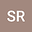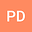Theoretical Prospects of the Solutions of Fractional Order Weakly Singular Volterra Integro Differential Equations and their Approximations with Convergence Analysis
••• Subrata Rana,
• Pratibhamoy Das
Subrata Rana
Indian Institute of Technology, Patna-801103, Bihar, INDIA

Corresponding Author:[email protected]

Author ProfilePratibhamoy Das
Indian Institute of Technology Patna Department of Mathematics
Author Profile## Abstract

In this work, we study a weakly singular Volterra integro differential equation with Caputo type fractional derivative. First, we derive a sufficient condition for the existence and uniqueness of the solution of this problem based on the maximum norm. It is observed that the condition depends on the domain of definition of the problem. Thereafter, we show that this condition will be independent of the domain of definition based on an equivalent weighted maximum norm. In addition, we have also provided a procedure to extend the existence and uniqueness of the solution in its domain of definition by partitioning it. We also derive a sufficient condition under which the model problem will provide an analytic solution. Next, we introduce a operator based parameterized method to generate an approximate solution of this problem. Convergence analysis of this approach is established here. Next, we have optimized this solution based on least square method. For this, residual minimization is used to obtain the optimal values of the auxiliary parameter. In addition, we have also provided an error bound based on this technique. Several numerical examples are produced to clarify the effective behavior of the convergence of the present method. Comparison of the standard method and optimized method based on residual minimization signify the better accuracy of modified one. In addition, we also consider an equivalent form of weakly singular integro differential equation of a Heat transfer problem to show the effectiveness of the present approach.
26 Feb 2020Submitted to Mathematical Methods in the Applied Sciences
01 Mar 2020Submission Checks Completed
01 Mar 2020Assigned to Editor
22 Mar 2020Reviewer(s) Assigned
09 Jul 2020Review(s) Completed, Editorial Evaluation Pending
10 Jul 2020Editorial Decision: Revise Major